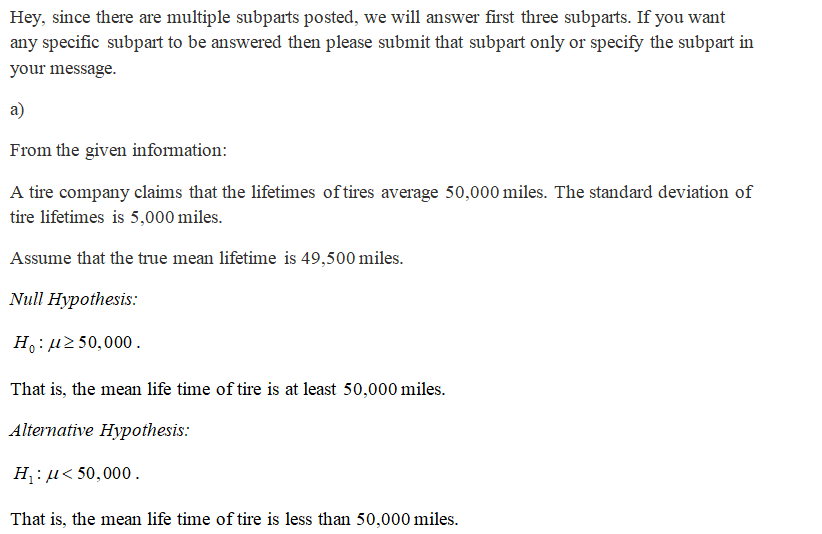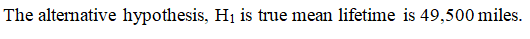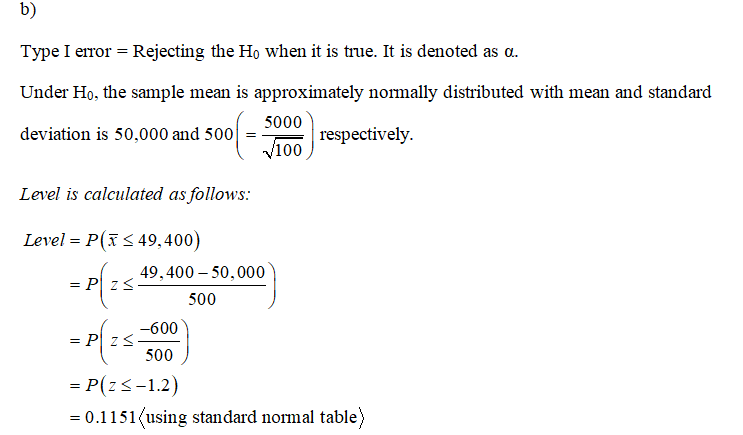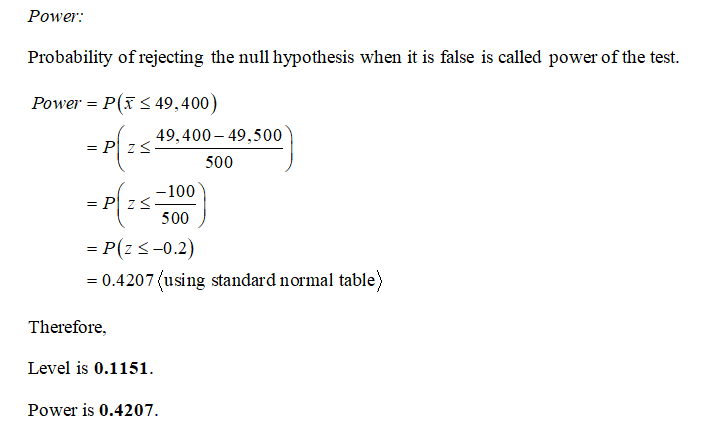# A tire company claims that the lifetimes of its tires average 50,000 miles. The standard deviation of tire lifetimes is known to be 5000 miles. You sample 100 tires and will test the hypothesis that the mean tire lifetime is at least 50,000 miles against the alternative that it is less. Assume, in fact, that the true mean lifetime is 49,500 miles. a) State the null and alternate hypotheses. Which hypothesis is true? b) It is decided to reject H0 if the sample mean is less than 49,400. Find the level and power of this test. c) If the test is made at the 5% level, what is the power? d) At what level should the test be conducted so that the power is 0.80? e) You are given the opportunity to sample more tires. How many tires should be sampled in total so that the power is 0.80 if the test is made at the 5% level?

Question
2 views

A tire company claims that the lifetimes of its tires average 50,000 miles. The standard deviation of tire lifetimes is known to be 5000 miles. You sample 100 tires and will test the hypothesis that the mean tire lifetime is at least 50,000 miles against the alternative that it is less. Assume, in fact, that the true mean lifetime is 49,500 miles. a) State the null and alternate hypotheses. Which hypothesis is true? b) It is decided to reject H0 if the sample mean is less than 49,400. Find the level and power of this test. c) If the test is made at the 5% level, what is the power? d) At what level should the test be conducted so that the power is 0.80? e) You are given the opportunity to sample more tires. How many tires should be sampled in total so that the power is 0.80 if the test is made at the 5% level?

check_circle

Step 1Step 2...

### Want to see the full answer?

See Solution

#### Want to see this answer and more?

Solutions are written by subject experts who are available 24/7. Questions are typically answered within 1 hour.*

See Solution
*Response times may vary by subject and question.
Tagged in